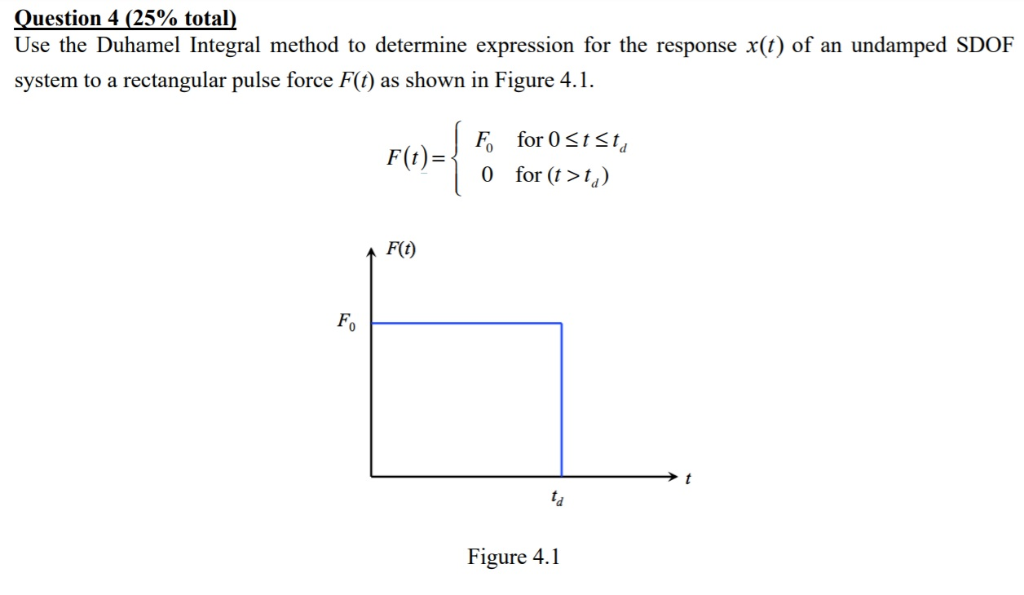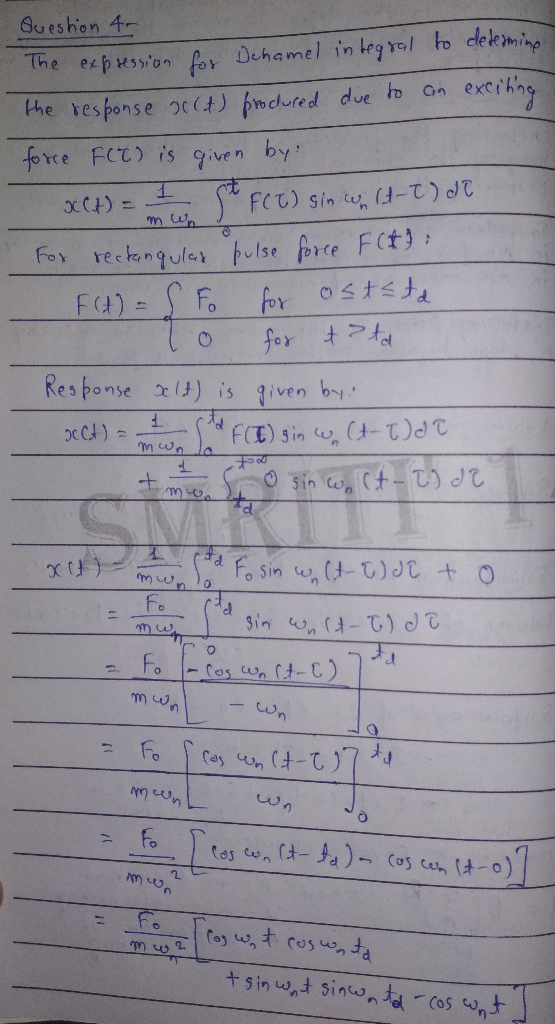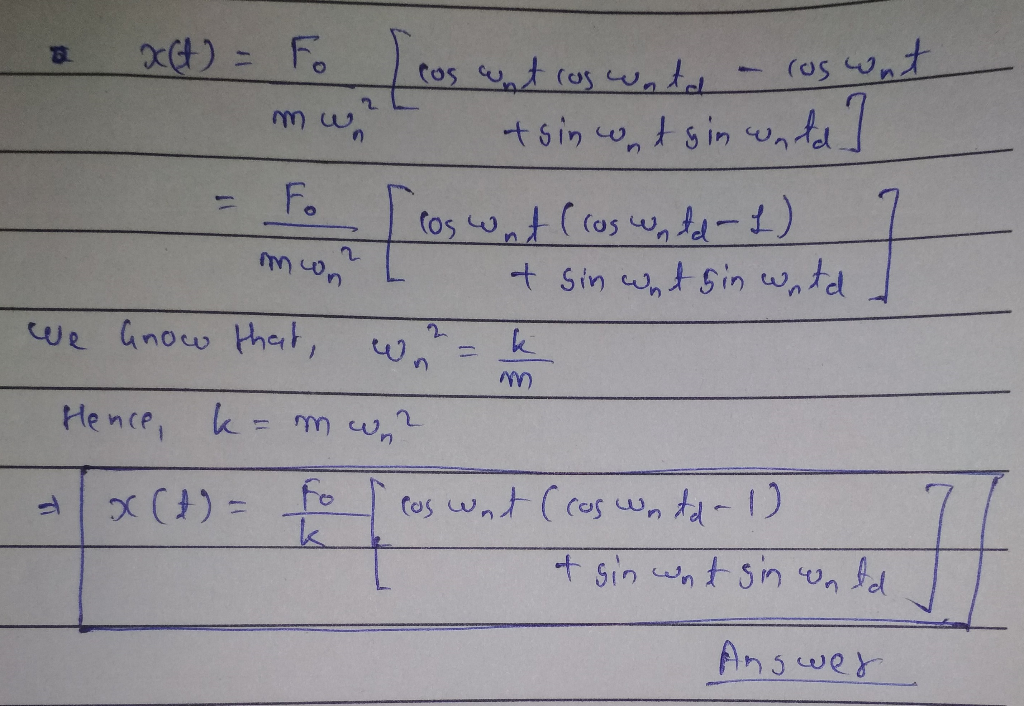# Question 4 (25% total) Use the Duhamel Integral method to determine expression for the response x(t) of an undamped SDO...Question 4 (25% total) Use the Duhamel Integral method to determine expression for the response x(t) of an undamped SDOF system to a rectangular pulse force F(t) as shown in Figure 4.1 F for O StSt Ffor («>) F(t) Fo Figure 4.1##### Add Answer of: Question 4 (25% total) Use the Duhamel Integral method to determine expression for the response x(t) of an undamped SDO...
More Homework Help Questions Additional questions in this topic.